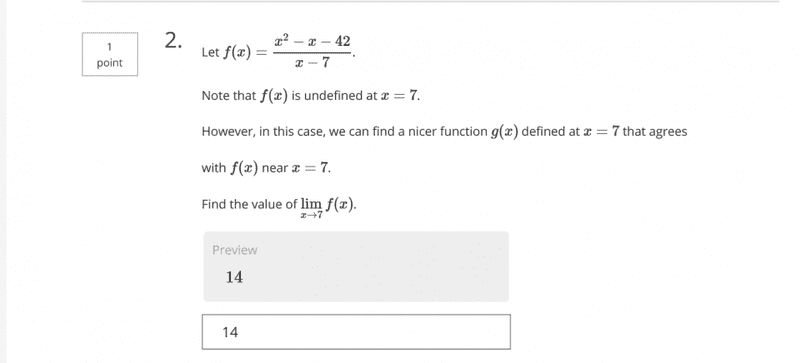# Stuck Again : -->I was doin' some coursera quiz. It asked this question :
Let ƒ(x) = (x2 - x - 42) / x-7
find as x → 7.
Now I approach x from both sides and it get me 2 different answer.
So which one is correct:
13 or 14?

haruspex
Homework Helper
Gold Member
View attachment 102575 I was doin' some coursera quiz. It asked this question :
Let ƒ(x) = (x2 - x - 42) / x-7
find as x → 7.
Now I approach x from both sides and it get me 2 different answer.
So which one is correct:
13 or 14?

SteamKing
Staff Emeritus
Homework Helper

symbolipoint
Homework Helper
Gold Member
Note that simplifying f will give the LINE y=x+6, and the FUNCTION is undefined for x=7. The limit at x=7 is that missing point. You just need to set x=7 for y=x+6.

OK get It.

Ray Vickson
Homework Helper
Dearly Missed
View attachment 102575 I was doin' some coursera quiz. It asked this question :
Let ƒ(x) = (x2 - x - 42) / x-7
find as x → 7.
Now I approach x from both sides and it get me 2 different answer.
So which one is correct:
13 or 14?

The function is not ##f(x) = \frac{x^2-x-42}{x} -7##, as you wrote.

The function is not ##f(x) = \frac{x^2-x-42}{x} -7##, as you wrote.
Yes it must be like ƒ(x) = (x2 - x - 42) / (x-7).